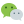# JavaScript 数组排序详解

``````var arr = [3, 1, 2];

console.log(arr.sort());
// [1, 2, 3]
console.log(arr); // sort() 函数会修改原数组
// [1, 2, 3]

arr = ['c', 'b','B', 'a','A'];
arr.sort();
console.log(arr);
// ["A", "B", "a", "b", "c"]
``````

``````var arr = [1, 2, 11, 12];
arr.sort();
console.log(arr);
// [1, 11, 12, 2]
``````

## 比较函数

• 如果 `compareFunction(a, b)` 返回值小于 0 ，那么 `a` 会被排列到 `b` 之前；
• 如果 `compareFunction(a, b)` 返回值等于 0 ，那么 `a``b` 的相对位置不变；
• 备注： ECMAScript 标准并不保证这一行为，而且也不是所有浏览器都会遵守（例如 Mozilla 在 2003 年之前的版本）；
• 如果 `compareFunction(a, b)` 返回值大于 0 ，那么 `b` 会被排列到 `a` 之前；

`compareFunction(a, b)` 必须总是对相同的输入返回相同的比较结果，否则排序的结果将是不确定的。

``````var arr = [2, 1, 4, 3];
arr.sort(function(a, b) {
console.log(a, b);
})

// 1 2
// 4 1
// 3 4
``````

## 升序

``````var arr = [2, 1, 3, 11, 12, 11]
arr.sort(function(a, b) {
return a - b;
})

console.log(arr);
// [1, 2, 3, 11, 11, 12]
``````

• 如果后一个元素比前一个元素小，即 `a - b < 0`，按照规则就是 `a` 要排到 `b` 的前面，也就是这两个元素会交换，小的在前，大的在后；
• 如果后一个元素比前一个元素大，即 `a - b > 0`，按照规则就是 `b` 要排到 `a` 的前面，由于 `b` 本来就在 `a` 的前面，所以两元素位置不变；
• 如果后一个元素与前一个元素相同，即 `a - b = 0`，按照规则就是 `a``b` 的位置不变，两元素位置同样不变；

## 降序

``````var arr = [2, 1, 3, 11, 12, 11]
arr.sort(function(a, b) {
return b - a;
})

console.log(arr);
// [12, 11, 11, 3, 2, 1]
``````

• 如果前一个元素比后一个元素小，即 `b - a < 0`，按照规则就是 `a` 要排到 `b` 的前面，也就是这两个元素会交换，大的在前，小的在后；
• 如果前一个元素比后一个元素大，即 `b - a > 0`，按照规则就是 `b` 要排到 `a` 的前面，由于 `b` 本来就在 `a` 的前面，所以两元素位置不变；
• 如果前一个元素与后一个元素相同，即 `b - a = 0`，按照规则就是 `a``b` 的位置不变，两元素位置同样不变；

## 反序

``````var arr = [2, 1, 4, 3];
arr.sort(function(a, b) {
return -1
});

console.log(arr);
// [3, 4, 1, 2]
``````

## 乱序

``````var arr = [1, 2, 3, 4, 5];
arr.sort((a, b) => {
return 0.5 - Math.random();
});

console.log(arr);
// [4, 3, 2, 1, 5]

arr.sort((a, b) => {
return 0.5 - Math.random();
});

console.log(arr);
// [5, 3, 1, 2, 4]
``````

### 评论：微信公众号：程序骑士